# Algebra 1 : How to multiply monomial quotients

## Example Questions

### Example Question #4783 : Algebra 1

Multiply the following monomial quotients: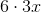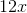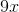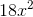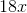Explanation:

To solve this problem, split it into two steps:

1. Multiply the coefficients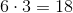2. Combine this number with the single variable to get the final answer: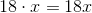### Example Question #4784 : Algebra 1

Multiply the following monomial quotients: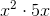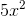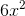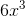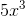Explanation:

To solve this problem, split it into two steps:

1. Multiply the coefficients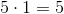2. Multiply the variables. We also need to remember the following laws of exponents rule: When multiplying variables, add the exponents.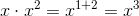Combine these to get the final answer:### Example Question #4785 : Algebra 1

Multiply the following monomial quotients: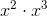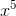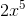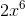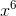Explanation:

To solve this problem, split it into two steps:

1. Multiply the coefficients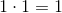2. Multiply the variables. We also need to remember the following laws of exponents rule: When multiplying variables, add the exponents.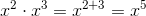Combine these to get the final answer:### Example Question #4786 : Algebra 1

Multiply the following monomial quotients: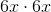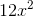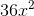Explanation:

To solve this problem, split it into two steps:

1. Multiply the coefficients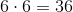2. Multiply the variables. We also need to remember the following laws of exponents rule: When multiplying variables, add the exponents.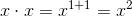Combine these to get the final answer:### Example Question #4787 : Algebra 1

Multiply the following monomial quotients: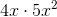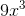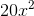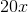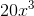Explanation:

To solve this problem, split it into two steps:

1. Multiply the coefficients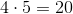2. Multiply the variables. We also need to remember the following laws of exponents rule: When multiplying variables, add the exponents.Combine these to get the final answer:### Example Question #4788 : Algebra 1

Multiply the following monomial quotients: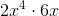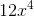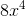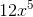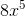Explanation:

To solve this problem, split it into two steps:

1. Multiply the coefficients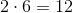2. Multiply the variables. We also need to remember the following laws of exponents rule: When multiplying variables, add the exponents.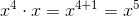Combine these to get the final answer:### Example Question #4789 : Algebra 1

Multiply the following monomial quotients: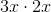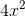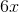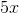Explanation:

To solve this problem, split it into two steps:

1. Multiply the coefficients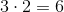2. Multiply the variables. We also need to remember the following laws of exponents rule: When multiplying variables, add the exponents.Combine these to get the final answer:### Example Question #4790 : Algebra 1

Multiply the following monomial quotients: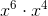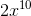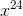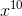Explanation:

To solve this problem, split it into two steps:

1. Multiply the coefficients2. Multiply the variables. We also need to remember the following laws of exponents rule: When multiplying variables, add the exponents.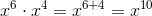Combine these to get the final answer:### Example Question #21 : How To Multiply Monomial Quotients

Multiply the following monomial quotients: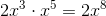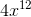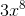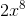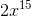Explanation:

To solve this problem, split it into two steps:

1. Multiply the coefficients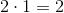2. Multiply the variables. We also need to remember the following laws of exponents rule: When multiplying variables, add the exponents.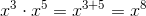Combine these to get the final answer:### Example Question #22 : How To Multiply Monomial Quotients

Multiply the following monomial quotients: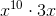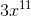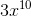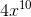Explanation:

To solve this problem, split it into two steps:

1. Multiply the coefficients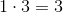2. Multiply the variables. We also need to remember the following laws of exponents rule: When multiplying variables, add the exponents.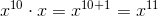Combine these to get the final answer: